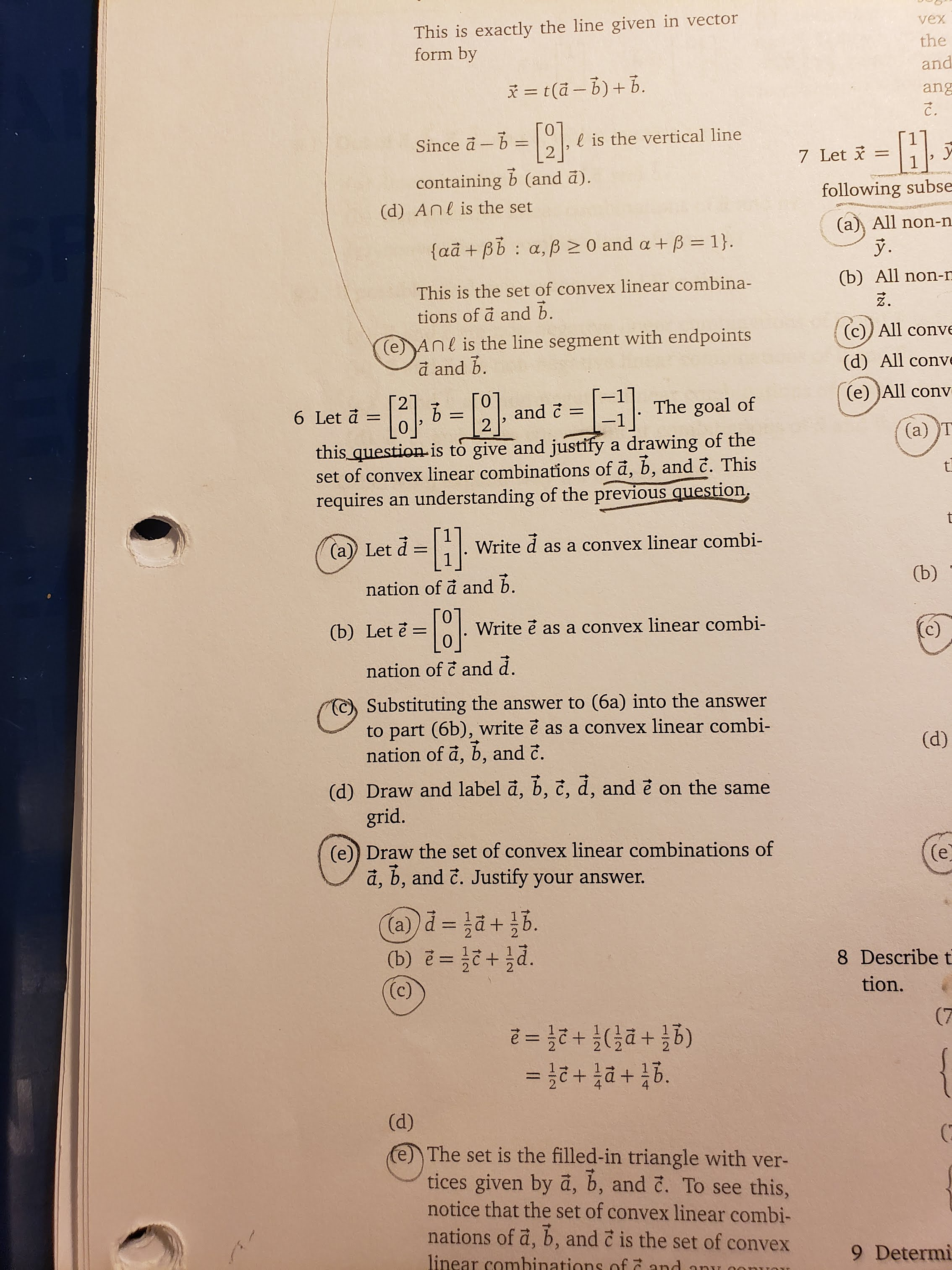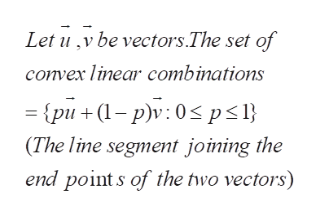This is exactly the line given in vectorform byvextheandt(a- b)b.ang-Hl is the vertical line2Since a b7 Letcontaining b (and a).following subse(d) Anl is the set(a All non-nfaã +Bb a, p0 and a +B = 1}Ў.(b) All non-nThis is the set of convex linear combina-tions of a and b.(c)) All conve(e)Anl is the line segment with endpointsa and b.(d) All conve(e) All convThe goal ofand c26 Let a-1-(a) Tthis question-is to give and justify a drawing of theset of convex linear combinations of a, b, and c. Thisrequires an understanding of the previous questiont-}(a)) LetWriteas a convex linear combi-(b)nation of a and b.(b) Let ě =Write e as a convex linear combi-(c)nation of c and d.Substituting the answer to (6a) into the answerto part (6b), write e as a convex linear combination of a, b, and c.(d)(d) Draw and label a, b, č, d, and e on the samegrid.(e)) Draw the set of convex linear combinations ofa, b, and c. Justify your answer.(e(a)+hd.(b) e=8 Describe t(c)tion.(7(g들 + D든)루 + 2든 =D2+(d)e The set is the filled-in triangle with ver-tices given by a, b, and č. To see this,notice that the set of convex linear combi-nations of a, b, and c is the set of convexlinear comhinations of and9 DetermiantC

Question

This problem is from Linear Algebra. It's based on Module 2. The book is called Linear Algebra Math Workbook 282 by Jason Siefkenhelp_outlineImage TranscriptioncloseThis is exactly the line given in vector form by vex the and t(a- b)b. ang -H l is the vertical line 2 Since a b 7 Let containing b (and a). following subse (d) Anl is the set (a All non-n faã +Bb a, p0 and a +B = 1} Ў. (b) All non-n This is the set of convex linear combina- tions of a and b. (c)) All conve (e)Anl is the line segment with endpoints a and b. (d) All conve (e) All conv The goal of and c 2 6 Let a - 1 - (a) T this question-is to give and justify a drawing of the set of convex linear combinations of a, b, and c. This requires an understanding of the previous question t -} (a)) Let Write as a convex linear combi- (b) nation of a and b. (b) Let ě = Write e as a convex linear combi- (c) nation of c and d. Substituting the answer to (6a) into the answer to part (6b), write e as a convex linear combi nation of a, b, and c. (d) (d) Draw and label a, b, č, d, and e on the same grid. (e)) Draw the set of convex linear combinations of a, b, and c. Justify your answer. (e (a) +hd. (b) e= 8 Describe t (c) tion. (7 (g들 + D든)루 + 2든 =D2 + (d) e The set is the filled-in triangle with ver- tices given by a, b, and č. To see this, notice that the set of convex linear combi- nations of a, b, and c is the set of convex linear comhinations of and 9 Determi an tC fullscreen
Step 1

The problem concerns convex linear combinations of vectors

Step 2

Similarly, if three vectors (non collinear) u, v and w are given, then the set of convex linear combinations consists of pu+qv+rw, with p,q,r lying between 0 a...help_outlineImage TranscriptioncloseLet uv be vectors.The set of convex linear combinations {pu(p)v 0

Want to see the full answer?

See Solution

Want to see this answer and more?

Our solutions are written by experts, many with advanced degrees, and available 24/7

See Solution
Tagged in

Math